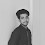# URI / BEE CROWD 1096 - Sequence IJ 2 Solution in C,C++,Python | URI - BEECROWD - BEE 1096 Solution in C,C++,Python

beecrowd | 1096

# Adapted by Neilor Tonin, URI  Brazil

Timelimit: 1

URI / BEE CROWD 1096 - Sequence IJ 2 Solution in C,C++,Python | URI - BEECROWD - BEE 1096 Solution in C,C++,Python:

Make a program that prints the sequence like the following example.

## Input

This problem doesn't have input.

## Output

Print the sequence like the example below.

 Input Sample Output Sample I=1 J=7I=1 J=6I=1 J=5I=3 J=7I=3 J=6I=3 J=5...I=9 J=7I=9 J=6I=9 J=5

Demonstration:

This program does not take user inputs . Then check the conditions for each test case contains two integer number i and j .Then calculating the sequence by using conditional loop.

Start with i =1 and j =7 . For every iteration, increment i by 2 and decrement j by 1.
Finally print the result.

N.B: Don't copy paste the code as same. Just try to understand it and try yourself.

## URI / BEECROWD Online Judge 1096 Solve in C :

```#include <stdio.h>

int main()
{
int I,J;
for(I=1;I<=9;I=I+2)
{
for(J=7;J>=5;J--)
printf ("I=%d J=%d\n",I,J);
}
return 0;
}```

### 2 Responses to URI / BEE CROWD 1096 - Sequence IJ 2 Solution in C,C++,Python | URI - BEECROWD - BEE 1096 Solution in C,C++,Python

1.Thank you for your work sir . you helped me alot

2.Welcome ❤️ also thanks for Supporting me 😇